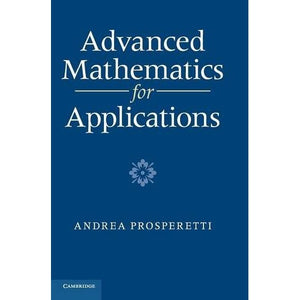>• £64.99
• Save £80

Andrea Prosperetti
Cambridge University Press, 1/6/2011
EAN 9780521515320, ISBN10: 0521515327

Hardcover, 744 pages, 24.7 x 17.4 x 4 cm
Language: English

The partial differential equations that govern scalar and vector fields are the very language used to model a variety of phenomena in solid mechanics, fluid flow, acoustics, heat transfer, electromagnetism and many others. A knowledge of the main equations and of the methods for analyzing them is therefore essential to every working physical scientist and engineer. Andrea Prosperetti draws on many years' research experience to produce a guide to a wide variety of methods, ranging from classical Fourier-type series through to the theory of distributions and basic functional analysis. Theorems are stated precisely and their meaning explained, though proofs are mostly only sketched, with comments and examples being given more prominence. The book structure does not require sequential reading: each chapter is self-contained and users can fashion their own path through the material. Topics are first introduced in the context of applications, and later complemented by a more thorough presentation.

Preface
List of tables
Part I. General Remarks and Basic Concepts
1. The classical field equations
2. Some simple preliminaries
Part II. Applications
3. Fourier series
applications
4. Fourier transform
applications
5. Laplace transform
applications
6. Cylindrical systems
7. Spherical systems
Part III. Essential Tools
8. Sequences and series
9. Fourier series
theory
10. The Fourier and Hankel transforms
11. The Laplace transform
12. The Bessel equation
13. The Legendre equation
14. Spherical harmonics
15. Green's functions
ordinary differential equations
16. Green's functions
partial differential equations
17. Analytic functions
18. Matrices and finite-dimensional linear spaces